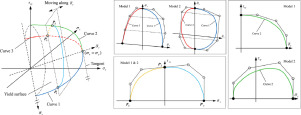International Journal of Mechanical Sciences ( IF 4.134 ) Pub Date : 2020-03-14 , DOI: 10.1016/j.ijmecsci.2020.105612
Sai Hao; Xianghuai DongIn this paper, the interpolation-type plane stress anisotropic yield models are proposed. The models are built in a specific three-dimensional oblique coordinate system by sequentially constructing three specific "longitude lines" and two sets of quasi-orthogonal "latitude lines" on the tricomponent yield surface. The three "longitude lines" act as the trajectories of the endpoints of the "latitude lines", and the semi-yield surface is formed by the continuous variation of the "latitude lines" along the trajectories. These curves are constructed by parametric Bézier curves, and different order schemes are adopted for “latitude lines” and “longitude lines”. Some coefficients in the expression of “latitude lines” are represented by specific analytic functions, which are related to the distortion of yield loci with the increase of τxy-component. According to whether the asymmetry of tension and compression when yielding is considered, two corresponding models are proposed. We also obtain corresponding simplified forms by omitting the parameter determination of the "latitude lines". The yield loci, flow stresses and r-valus of five kinds of materials with three lattice types were predicted by full-parameter models and simplified models, which showed the accuracy of the proposed models for predicting yield behavior of various materials.

down
wechat
bug